# subtract

Boolean subtraction operation on two RF PCB shapes

## Syntax

``c = subtract(shape1,shape2)``

## Description

example

````c = subtract(shape1,shape2)` subtracts `shape1` and `shape2` using the subtract operation. You can also use the `-` symbol to subtract the two shapes.```

## Examples

collapse all

Create and display a cross trace shape.

```trace = traceCross; show(trace)```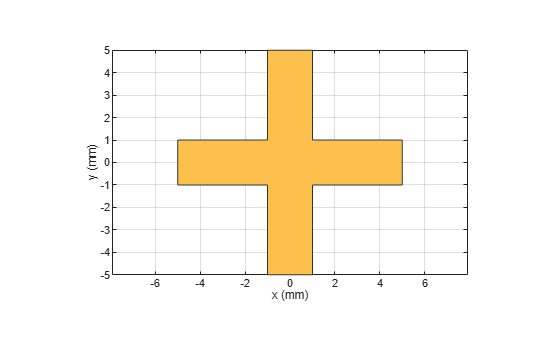Create and display an annular ring shape with an inner radius of 4 m.

```ring = ringAnnular(InnerRadius=4); show(ring)```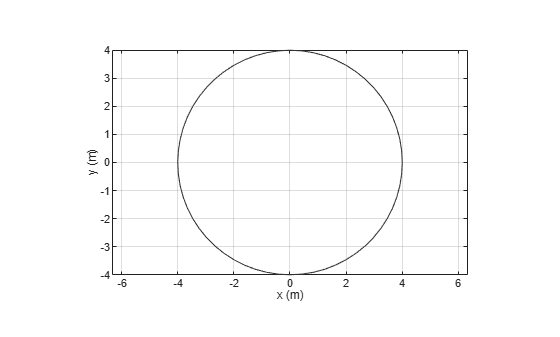Subtract the annular ring from the cross trace and display the result.

```shapeDiff = subtract(trace,ring); show(shapeDiff)```Create and display a curved bend shape.

```bend1 = bendCurved; show(bend1)```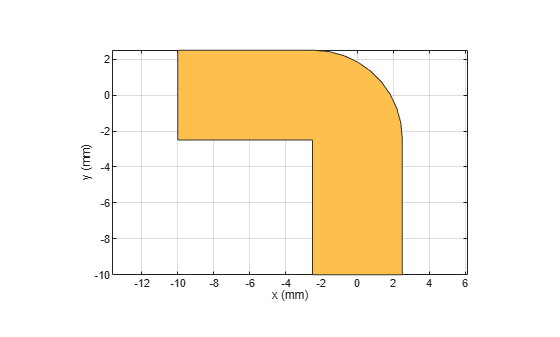Create and display a curved bend shape with a spatial offset.

```bend2 = bendCurved(ReferencePoint=[-1e-3 -1e-3]); show(bend2)```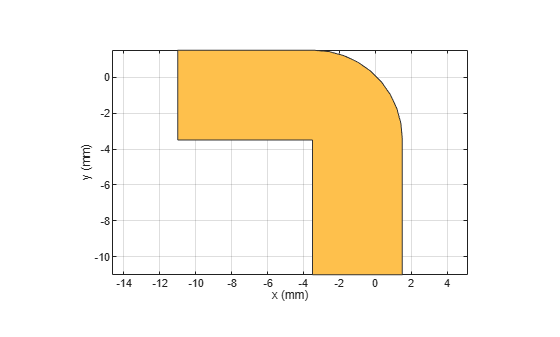Subtract the offset bend from the default bend and display the result.

```shapeDiff = bend1 - bend2; show(shapeDiff)```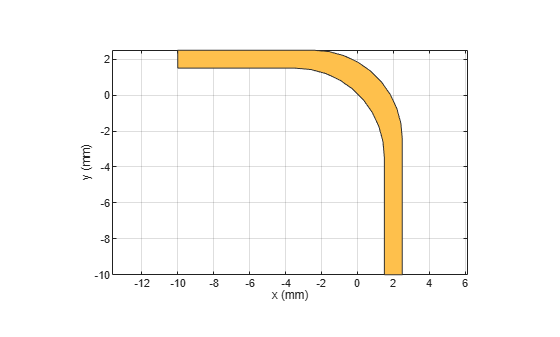## Input Arguments

collapse all

First shape created using custom elements and shape objects of RF PCB Toolbox™, specified as an object.

Example: `shape1 = bendCurved;` specifies the first shape as a `bendCurved` object.

Second shape created using custom elements and shape objects of RF PCB Toolbox, specified as an object.

Example: `shape2 = ringAnnular;` specifies the second shape as a `ringAnnular` object.

## Version History

Introduced in R2021b